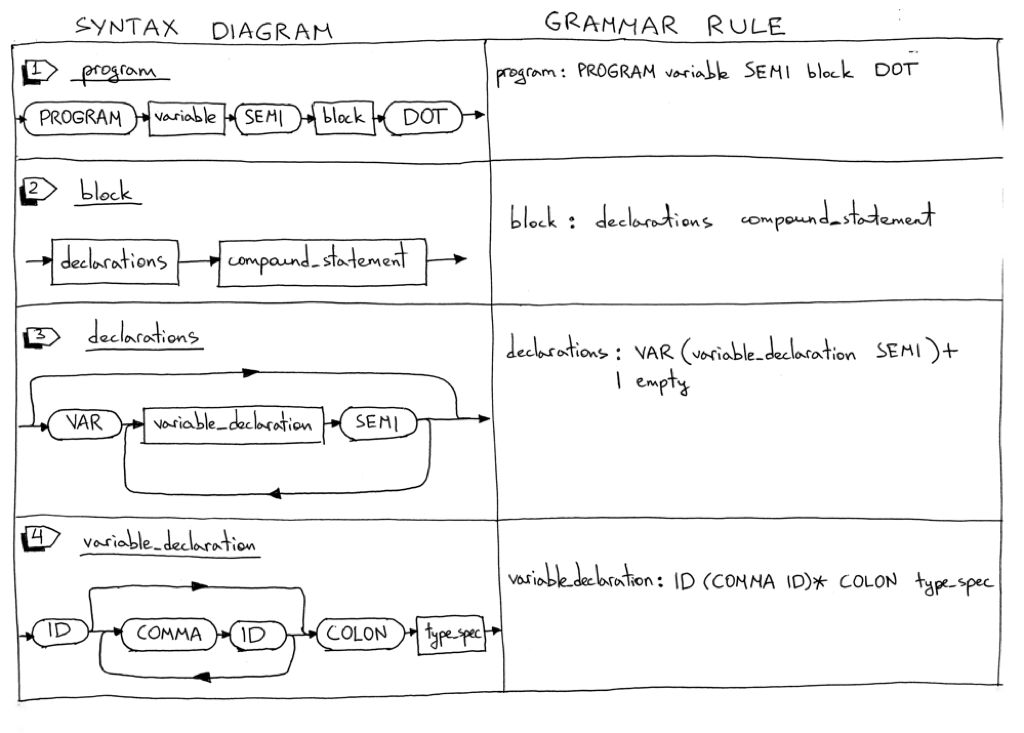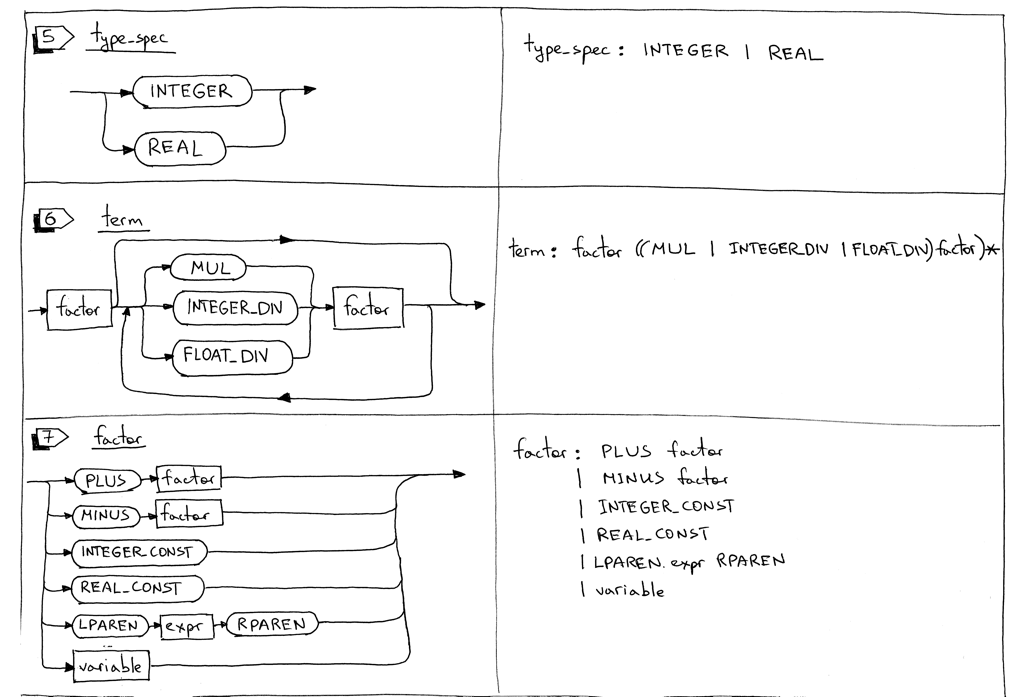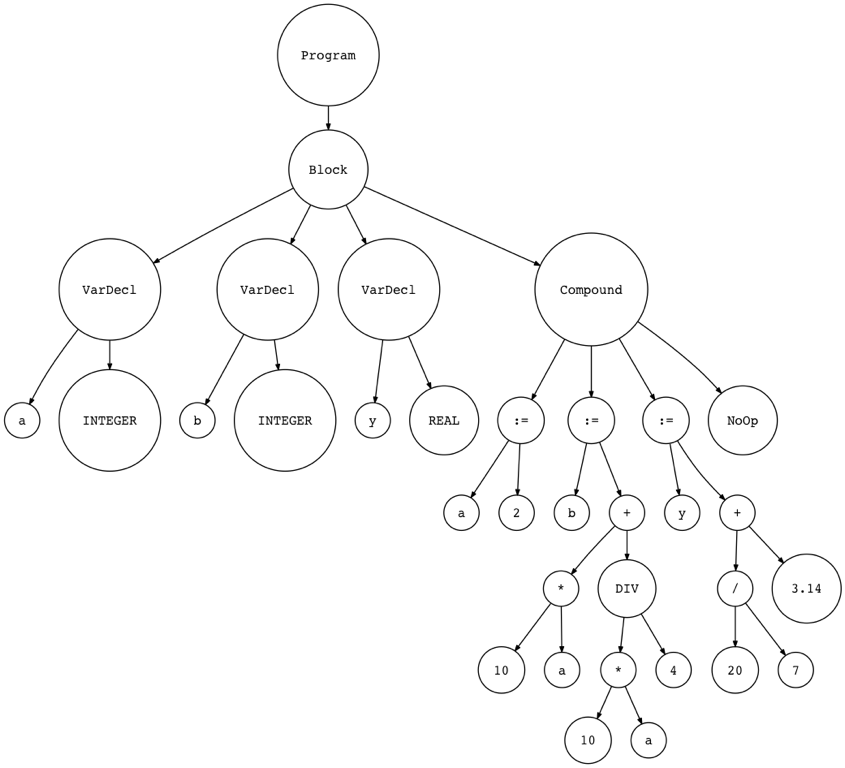# 一起来写个简单的解释器（10）``````PROGRAM Part10;
VAR
number     : INTEGER;
a, b, c, x : INTEGER;
y          : REAL;

BEGIN {Part10}
BEGIN
number := 2;
a := number;
b := 10 * a + 10 * number DIV 4;
c := a - - b
END;
x := 11;
y := 20 / 7 + 3.14;
{ writeln('a = ', a); }
{ writeln('b = ', b); }
{ writeln('c = ', c); }
{ writeln('number = ', number); }
{ writeln('x = ', x); }
{ writeln('y = ', y); }
END.  {Part10}
``````

``````\$ python spi.py part10.pas
a = 2
b = 25
c = 27
number = 2
x = 11
y = 5.99714285714
``````

``````\$ fpc part10.pas
\$ ./part10
a = 2
b = 25
c = 27
number = 2
x = 11
y =  5.99714285714286E+000
``````

1. 学习如何解析和解释Pascal PROGRAM
2. 学习如何解析Pascal变量声明
3. 更新解释器，使用DIV关键字进行整数除法，使用正斜杠 / 进行浮点除法
4. 增加对Pascal评论的支持1. program定义语法规则被更新以包括PROGRAM保留关键字、程序名称和以点结尾的程序块。下面是一个完整的Pascal程序的例子： ``` PROGRAM Part10; BEGIN END. ```
2. block规则包含了一个declarations规则和一个compound_statement规则。当我们增加过程声明时，我们也会使用这个规则。这是一个block的例子：

``````VAR
number : INTEGER;

BEGIN
END
``````

这是另一个例子：

``````BEGIN
END
``````
3. Pascal的声明有几个部分，每个部分都是可选的。在这篇文章中，我们只会介绍变量声明部分。declarations 规则或者有一个变量声明子规则或者为空。

4. Pascal是一种静态类型的语言，这意味着每个变量都需要一个变量声明来显式的指定它的类型。在Pascal中，变量必须在使用之前声明。这是通过使用VAR保留关键字在程序变量声明部分中声明变量来实现的。你可以像这样定义变量：

``````VAR
number     : INTEGER;
a, b, c, x : INTEGER;
y          : REAL;
``````
5. type_spec规则用于处理INTEGER和REAL类型，用于变量声明中。在下面的例子中

``````VAR
a : INTEGER;
b : REAL;
``````

变量“a”用INTEGER类型声明，变量“b”用REAL（float）类型声明。在本文中，我们不会强制进行类型检查，但是我们将在本系列的后面添加类型检查。

6. term规则更新为使用DIV关键字进行整数除法和正斜杠 / 进行浮点除法。

之前，20除7使用正斜杠将产生一个INTEGER 2：

``````20 / 7 = 2
``````

现在，20除以7使用正斜杠将产生一个REAL（浮点数）2.85714285714：

``````20 / 7 = 2.85714285714
``````

从现在开始，要获得INTEGER而不是REAL，您需要使用DIV关键字：

``````20 DIV 7 = 2
``````
7. factor规则被更新来处理整数和实数（float）常量。我还删除了INTEGER子规则，因为常量将由INTEGER_CONST和REAL_CONST标记表示，而INTEGER标记将用于表示整数类型。在下面的例子中，词法分析器将为20和7生成一个INTEGER_CONST标记，为3.14生成一个REAL_CONST标记：

``````y := 20 / 7 + 3.14;
``````

``````program : PROGRAM variable SEMI block DOT

block : declarations compound_statement

declarations : VAR (variable_declaration SEMI)+
| empty

variable_declaration : ID (COMMA ID)* COLON type_spec

type_spec : INTEGER

compound_statement : BEGIN statement_list END

statement_list : statement
| statement SEMI statement_list

statement : compound_statement
| assignment_statement
| empty

assignment_statement : variable ASSIGN expr

empty :

expr : term ((PLUS | MINUS) term)*

term : factor ((MUL | INTEGER_DIV | FLOAT_DIV) factor)*

factor : PLUS factor
| MINUS factor
| INTEGER_CONST
| REAL_CONST
| LPAREN expr RPAREN
| variable

variable: ID
``````

1. 更新词法分析器
2. 更新解析器
3. 更新解释器

1. 新的标记
2. 新的和更新的保留关键字
4. 重命名integer方法，并对方法本身进行一些更改
5. 更新get_next_token方法以返回新的标记

1. 为了处理程序头，变量声明，整数和浮点常量以及整数和浮点除法，我们需要添加一些新的标记（其中一些是保留的关键字），我们还需要更新INTEGER标记的含义来表示整数类型，而不是一个整数常量。以下是新的和更新的标记的完整列表：

• PROGRAM (保留关键字)
• VAR (保留关键字)
• COLON (:)
• COMMA (,)
• INTEGER (我们将其更改为表示整数类型，而不是像3或5那样的整数常量)
• REAL(对应Pascal REAL类型)
• INTEGER_CONST(例如3或5)
• REAL_CONST(例如3.14等)
• INTEGER_DIV用于整数除法 (DIV保留关键字)
• FLOAT_DIV浮点除法 (正斜杠/)
2. 以下是保留关键字到标记的完整映射：

``````RESERVED_KEYWORDS = {
'PROGRAM': Token('PROGRAM', 'PROGRAM'),
'VAR': Token('VAR', 'VAR'),
'DIV': Token('INTEGER_DIV', 'DIV'),
'INTEGER': Token('INTEGER', 'INTEGER'),
'REAL': Token('REAL', 'REAL'),
'BEGIN': Token('BEGIN', 'BEGIN'),
'END': Token('END', 'END'),
}
``````
3. 增加skip_comment方法来处理Pascal注释。该方法非常基础，它所做的就是忽略所有字符，直到找到闭合大括号。

``````def skip_comment(self):
while self.current_char != '}':
self.advance()  # the closing curly brace
``````
4. 重命名integer方法为number方法。 它可以同时处理整数常量和浮点常量，如3和3.14：

``````def number(self):
"""Return a (multidigit) integer or float consumed from the input."""
result = ''
while self.current_char is not None and self.current_char.isdigit():
result += self.current_char

if self.current_char == '.':
result += self.current_char

while (
self.current_char is not None and
self.current_char.isdigit()
):
result += self.current_char

token = Token('REAL_CONST', float(result))
else:
token = Token('INTEGER_CONST', int(result))

``````
5. 更新get_next_token方法来返回新的标记：

``````def get_next_token(self):
while self.current_char is not None:
...
if self.current_char == '{':
self.skip_comment()
continue
...
if self.current_char.isdigit():
return self.number()

if self.current_char == ':':

if self.current_char == ',':
...
if self.current_char == '/':
...
``````

1. 新的AST节点：Program, Block, VarDecl, Type
2. 对应于新语法规则的新方法：block, declarations, variable_declaration, 和 type_spec.
3. 更新现有的解析器方法： program, term, 和 factor

1. 我们将首先从新的AST节点开始。有四个新节点：

• Program AST节点代表一个程序，将成为我们的根节点

``````class Program(AST):
def __init__(self, name, block):
self.name = name
self.block = block
``````
• Block AST节点包含声明和复合语句：

``````class Block(AST):
def __init__(self, declarations, compound_statement):
self.declarations = declarations
self.compound_statement = compound_statement
``````
• VarDecl AST节点表示一个变量声明。它包含一个变量节点和一个类型节点：

``````class VarDecl(AST):
def __init__(self, var_node, type_node):
self.var_node = var_node
self.type_node = type_node
``````
• Type AST节点表示变量类型（INTEGER或REAL）：

``````class Type(AST):
def __init__(self, token):
self.token = token
self.value = token.value
``````
2. 正如您记的那样，语法中的每个规则在我们的递归下降解析器中都有相应的方法。今天，我们添加四个新的方法：block, declarations, variable_declaration, 和 type_spec。这些方法负责解析新的语言结构和构建新的AST节点：

``````def block(self):
"""block : declarations compound_statement"""
declaration_nodes = self.declarations()
compound_statement_node = self.compound_statement()
node = Block(declaration_nodes, compound_statement_node)
return node

def declarations(self):
"""declarations : VAR (variable_declaration SEMI)+
| empty
"""
declarations = []
if self.current_token.type == VAR:
self.eat(VAR)
while self.current_token.type == ID:
var_decl = self.variable_declaration()
declarations.extend(var_decl)
self.eat(SEMI)

return declarations

def variable_declaration(self):
"""variable_declaration : ID (COMMA ID)* COLON type_spec"""
var_nodes = [Var(self.current_token)]  # first ID
self.eat(ID)

while self.current_token.type == COMMA:
self.eat(COMMA)
var_nodes.append(Var(self.current_token))
self.eat(ID)

self.eat(COLON)

type_node = self.type_spec()
var_declarations = [
VarDecl(var_node, type_node)
for var_node in var_nodes
]
return var_declarations

def type_spec(self):
"""type_spec : INTEGER
| REAL
"""
token = self.current_token
if self.current_token.type == INTEGER:
self.eat(INTEGER)
else:
self.eat(REAL)
node = Type(token)
return node
``````
3. 我们还需要更新program、 term和factor方法以适应语法变化：

``````def program(self):
"""program : PROGRAM variable SEMI block DOT"""
self.eat(PROGRAM)
var_node = self.variable()
prog_name = var_node.value
self.eat(SEMI)
block_node = self.block()
program_node = Program(prog_name, block_node)
self.eat(DOT)
return program_node

def term(self):
"""term : factor ((MUL | INTEGER_DIV | FLOAT_DIV) factor)*"""
node = self.factor()

while self.current_token.type in (MUL, INTEGER_DIV, FLOAT_DIV):
token = self.current_token
if token.type == MUL:
self.eat(MUL)
elif token.type == INTEGER_DIV:
self.eat(INTEGER_DIV)
elif token.type == FLOAT_DIV:
self.eat(FLOAT_DIV)

node = BinOp(left=node, op=token, right=self.factor())

return node

def factor(self):
"""factor : PLUS factor
| MINUS factor
| INTEGER_CONST
| REAL_CONST
| LPAREN expr RPAREN
| variable
"""
token = self.current_token
if token.type == PLUS:
self.eat(PLUS)
node = UnaryOp(token, self.factor())
return node
elif token.type == MINUS:
self.eat(MINUS)
node = UnaryOp(token, self.factor())
return node
elif token.type == INTEGER_CONST:
self.eat(INTEGER_CONST)
return Num(token)
elif token.type == REAL_CONST:
self.eat(REAL_CONST)
return Num(token)
elif token.type == LPAREN:
self.eat(LPAREN)
node = self.expr()
self.eat(RPAREN)
return node
else:
node = self.variable()
return node
``````

``````PROGRAM Part10AST;
VAR
a, b : INTEGER;
y    : REAL;

BEGIN {Part10AST}
a := 2;
b := 10 * a + 10 * a DIV 4;
y := 20 / 7 + 3.14;
END.  {Part10AST}
``````

genastdot.py产生AST并观察它：

``````\$ python genastdot.py part10ast.pas > ast.dot && dot -Tpng -o ast.png ast.dot
``````• visit_Program
• visit_Block
• visit_VarDecl
• visit_Type

``````def visit_Program(self, node):
self.visit(node.block)

def visit_Block(self, node):
for declaration in node.declarations:
self.visit(declaration)
self.visit(node.compound_statement)

def visit_VarDecl(self, node):
# Do nothing
pass

def visit_Type(self, node):
# Do nothing
pass
``````

``````def visit_BinOp(self, node):
if node.op.type == PLUS:
return self.visit(node.left) + self.visit(node.right)
elif node.op.type == MINUS:
return self.visit(node.left) - self.visit(node.right)
elif node.op.type == MUL:
return self.visit(node.left) * self.visit(node.right)
elif node.op.type == INTEGER_DIV:
return self.visit(node.left) // self.visit(node.right)
elif node.op.type == FLOAT_DIV:
return float(self.visit(node.left)) / float(self.visit(node.right))
``````

• 将新规则添加到语法并更新一些现有的规则
• 向词法分析器添加新的标记和支持方法，更新和修改一些现有的方法
• 为新的语言结构在解析器添加新的AST节点
• 将与新语法规则对应的新方法添加到我们的递归下降分析器中，并更新一些现有的方法
• 向解释器添加新的访问者方法并更新现有的访问者方法

• 解释器现在可以处理PROGRAM头
• 现在可以使用VAR关键字来声明变量
• DIV关键字用于整数除法，正斜杠用于浮点除法